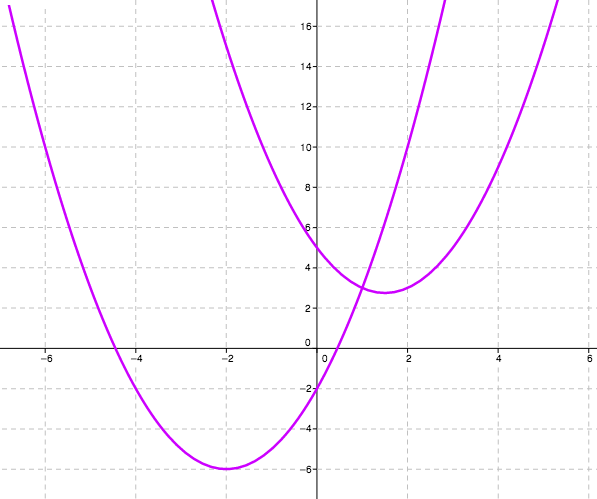### Home > AC > Chapter 12 > Lesson 12.3.1 > Problem12-59

12-59.

Where do the two parabolas below intersect? Show how you know.

$\begin{array}{c} y = x^2 + 4x - 2 \\ y = x^2 - 3x + 5 \end{array}$

Graph the parabolas. You can find where they intersect on the graph.Set the equations equal to each other. Solve for $x$.